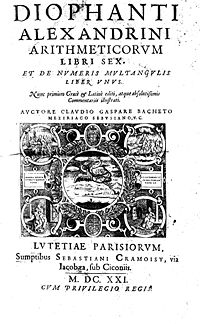Diophantus of Alexandria (dates unknown, lived and died between 200AD and 300AD) was an Alexandrian mathematician. He wrote a series of books (many now lost) called Arithmetica that deal with solving algebraic equations. Pierre de Fermat studied Arithmetica and it was in the margin in his copy that he made the fateful note in 1637 that a certain equation similar to the Pythagorean equation considered by Diophantus has no solutions, and he found "a truly marvelous proof of this proposition", the celebrated Fermat's Last Theorem. This led to tremendous advances in number theory, and the study of diophantine equations ("diophantine geometry") and of diophantine approximations remain important areas of mathematical research. Diophantus was the first Greek mathematician who recognized fractions as numbers; thus he allowed positive rational numbers for the coefficients and solutions.

In modern use, diophantine equations are usually algebraic equations with integer coefficients, for which integer solutions are sought.

One of the Famous People on this site.